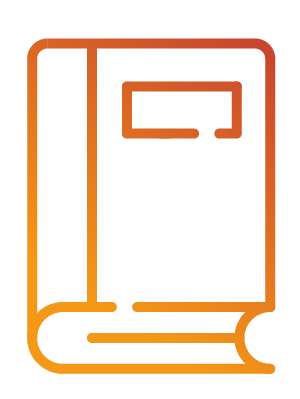Système d’information en philosophie des sciencesInformation system on philosophy of science

ImprimerEnvoyer le lien

MONOGRAPHIEThe Nature of Mathematics. A Critical Survey

Résumé :

Anglais

This book presents a considered critical exposition of Principia Mathematica and gives allied views on the nature of mathematics, with supplementary accounts of the Formalist and Intuitionist doctrines. The contributions of Chwistek, Ramsey, Wittgenstein, Weyl, and others to logistic theory are described and considered in relation to the work of Russell and Whitehead and the opposing continental schools, whose theories have been comparatively neglected by English-speaking commentators. The discussion of pure mathematics and the principles of symbolism incidental to the account should interest those concerned with the philosophical implications of mathematics, while mathematicians and others interested in the nature of mathematics will be able to find a not unnecessarily technical introduction to recent theories. – Introduction. – Preliminary survey of three types of opinions considered. – Logistic. – Formalism. – Intuitionism. – Mutual relations of the three schools. – Section I. Logistic : History of logistic views of mathematics; The tasks of a philosophy of mathematics; Suppementary note on logical analysis; The formal character of pure mathematics; The propositional calculus; The calculus of propositional functions; Variable and function in mathematics; Various usages of variable symbols; Definitions of mathematical functions; Propositional functions; The calculus of propositional functions resumed; Quantifiers, truth-values, etc.; The algebra of propositional functions; Extensional propositional functions; Propositional functions of functions and the general functional calculus; Extensional functions of functions; Derivation of mathematical functions from propositional descriptions; Plural descriptive phrases; Definitions of descriptions and classes; Complete and incomplete symbols; Definition of incomplete symbol; Importance of incomplete symbols; Ontological status of incomplete symbols; Nature of Principia classes; Consistency of definition of classes as incomplete symbols; The real number; Intuitions of continuity in a sensory field; Continuity in geometrical space; Dedekind’s definition of real number; The logistico-mathematical paradoxes; Solution of the paradoxes; Note on types and orders; Connection between the “extended infinite” and the paradoxes; Confusion of types in the theorem of the upper bound; The axiom of reducibility and the logistic definition of real number; The axiom of reducibility; The axiom of infinity; Arguments for the axiom of reducibility; Axiom of reducibility equivalent to the assertion of the existence of c propositional functions; Other criticisms of the axiom of reducibility; F. P. Ramsy; Note on the thesis of extensionality; H. Weyl; L. Wittgenstein; L. Chwistek; Conclusions. – Section II. Formalism : The development of geometry; The formalist view of mathematics; The formalist programme in detail; Note on Gödel’s theorem. – Section III. Intuitionism : The mathematical predecessors of the intuitionists; Digression on the theory of sets of points; The mathematical controversy; Intuitionism; Kant and Brouwer; The sociological basis of mathematics; The denial of the law of the excluded middle; The intuitionist continuum; Supplementary note on the intuitionist calculus of propositions; Intuitionist theory of cardinal numbers. M.-M. V.